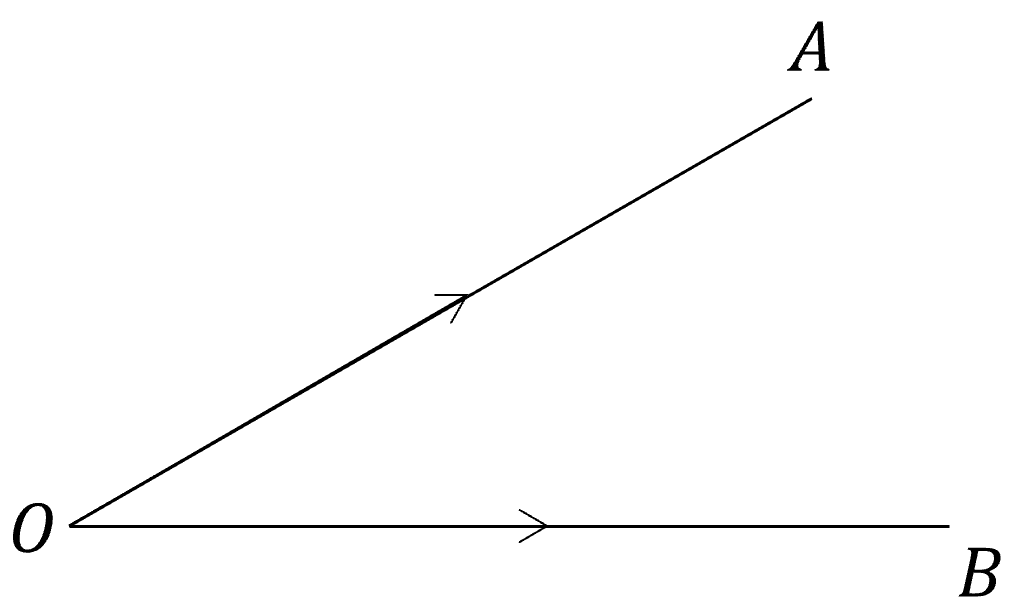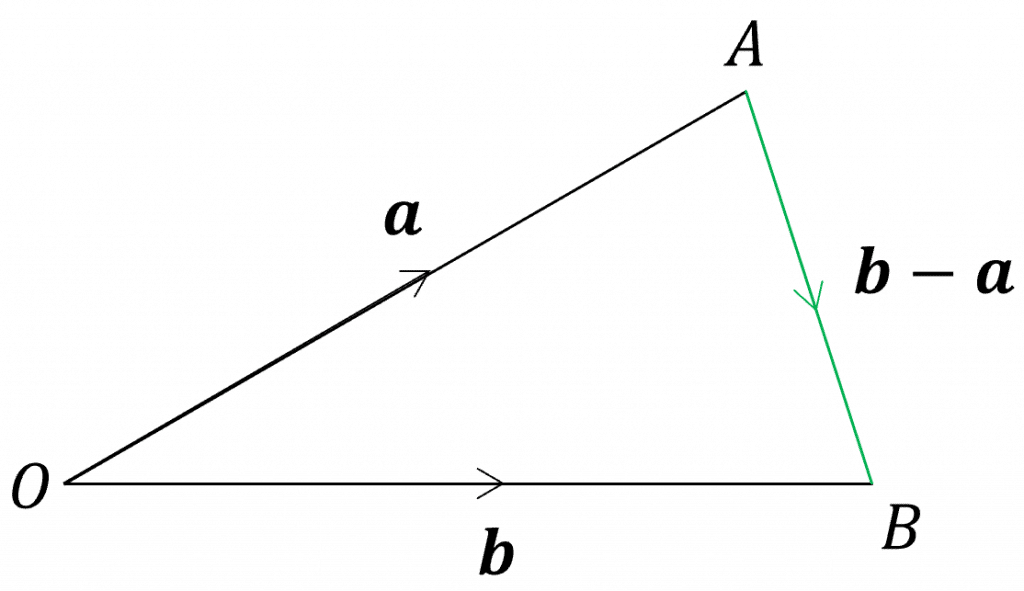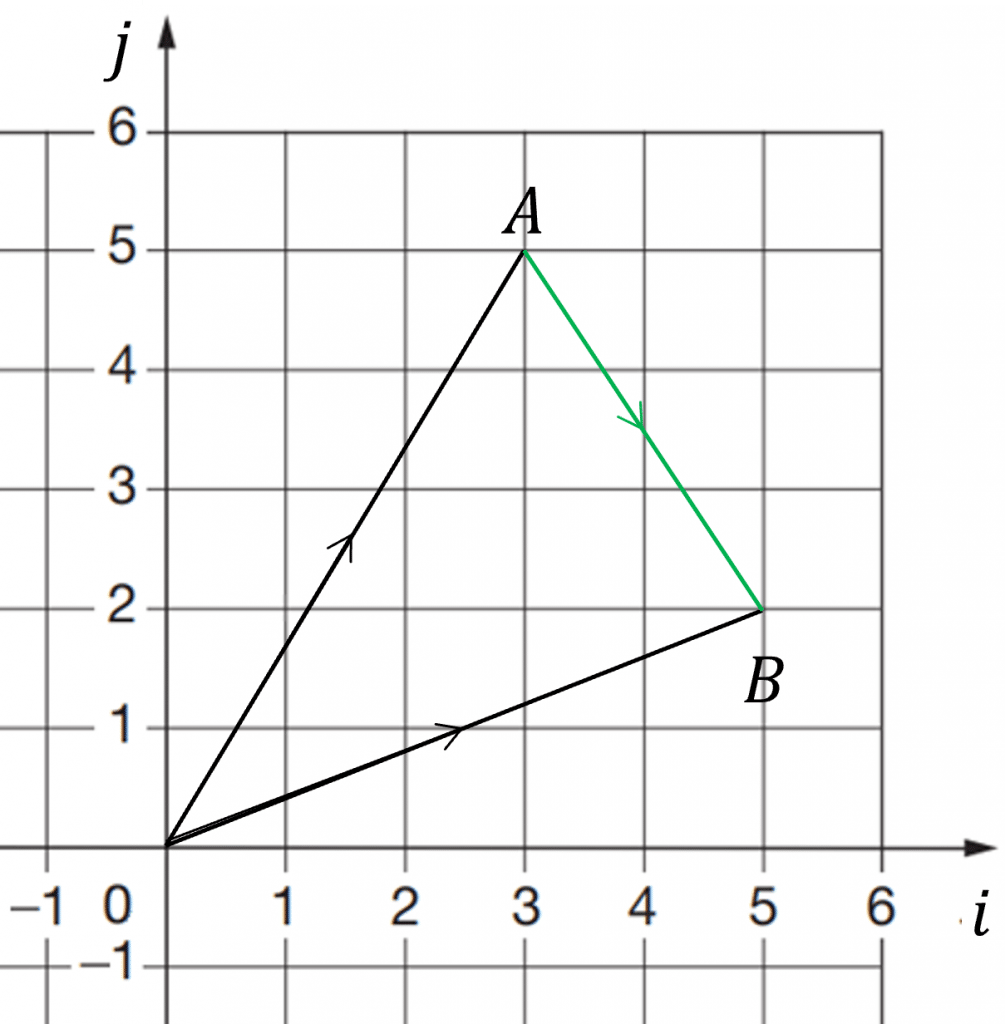# Position Vectors

A LevelAQAEdexcelOCR

## Position Vectors

A position vector describes where a point lies, in relation to the origin, $\boldsymbol{O}$

This allows you to write other vectors in terms of position vectors.

Make sure you are happy with the following topics before continuing.

A LevelAQAEdexcelOCR## Working with Position Vectors

For a point $A$, the position vector is denoted as $\overrightarrow{OA}$

Likewise the point $B$ is denoted as $\overrightarrow{OB}$Writing points as position vectors then allows you to describe vectors between points as position vectors.

If we let, $\boldsymbol{a}=\overrightarrow{OA}$ and $\boldsymbol{b}=\overrightarrow{OB}$

Then, $\overrightarrow{AB}=-\overrightarrow{OA}+\overrightarrow{OB}=-\boldsymbol{a}+\boldsymbol{b}=\boldsymbol{b}-\boldsymbol{a}$

A LevelAQAEdexcelOCR

## Describing vectors using $\boldsymbol{i}$ and $\boldsymbol{j}$ Units

• A vector with a magnitude of $1$ unit is called a unit vector
• The standard unit vectors are $\boldsymbol{i}$ and $\boldsymbol{j}$, which are in the direction of the $x$-axis and $y$-axis respectively.
• These standard unit vectors are used to express the horizontal and vertical position of the end of a vector compared to its start pointUsing the diagram on the right, we can see the position vector of point $A=3\boldsymbol{i}+5\boldsymbol{j}$ and the position vector of point $B=5\boldsymbol{i}+2\boldsymbol{j}$

To write $\overrightarrow{AB}$ in terms of standard unit vectors, we can just add and subtract the $\boldsymbol{i}$ and $\boldsymbol{j}$ components of $\boldsymbol{a}$ and $\boldsymbol{b}$ separately.

Thus the vector $\overrightarrow{AB}=-\boldsymbol{a}+\boldsymbol{b}=-(3\boldsymbol{i}+5\boldsymbol{j})+(5\boldsymbol{i}+2\boldsymbol{j})=2\boldsymbol{i}-3\boldsymbol{j}$

This means that to go from $A$ to $B$ you go $2$ units to the right and $3$ units down.

A LevelAQAEdexcelOCR

## Column Vectors

Column vectors are another way of expressing vectors.

If $A=4\boldsymbol{i}-3\boldsymbol{j}$, then the column vector for $A$ is $\dbinom{4}{-3}$

To add and subtract column vectors from one another you simply add or subtract the top row and then add or subtract the bottom row. For example, if $A=\dbinom{5}{-2}$ and $B=\dbinom{1}{3}$, then $A+B=\dbinom{5+1}{-2+3}=\dbinom{6}{1}$

Multiplying a column vector by a scalar is also straight forward, you just need to multiply both the top and bottom numbers by the scalar. For instance, if $C=\dbinom{-3}{4}$, $3C=\dbinom{3\times-3}{3\times4}=\dbinom{-9}{12}$

A LevelAQAEdexcelOCR

## Position Vectors Example Questions

If $A=(x,y)$, then $A=x\boldsymbol{i}+y\boldsymbol{j}=\dbinom{x}{y}$

So,

a) $K=-\boldsymbol{i}+3\boldsymbol{j}$

b) $K=\dbinom{-1}{3}$

$2\boldsymbol{a}-4\boldsymbol{b}=\dbinom{2\times7}{2\times-2}-\dbinom{4\times-3}{4\times-1}=\dbinom{14}{-4}-\dbinom{-12}{-4}=\dbinom{14-(-12)}{-4-(-4)}=\dbinom{26}{0}$
$\overrightarrow{XY}=\boldsymbol{y}-\boldsymbol{x}=(-3\boldsymbol{i}+4\boldsymbol{j})-(5\boldsymbol{i}-\boldsymbol{j})=-8\boldsymbol{i}+5\boldsymbol{j}$

We know that point $Z$ lies on the line $XY$, such that $XZ:ZY=2:3$, so $\overrightarrow{XZ}=\dfrac{2}{5}\overrightarrow{XY}$

Therefore we can calculate the position of point $Z$ from $X$:

$\overrightarrow{XZ}=\dfrac{2}{5}(-8\boldsymbol{i}+5\boldsymbol{j})=-\dfrac{16}{5}\boldsymbol{i}+2\boldsymbol{j}$

Finally, add the position vector of  $\overrightarrow{XZ}$ to the position vector of $X$ to get the position vector of $Z$ (from the origin):

$Z = 5\boldsymbol{i}-\boldsymbol{j}-\dfrac{16}{5}\boldsymbol{i}+2\boldsymbol{j}=\dfrac{9}{5}\boldsymbol{i}+\boldsymbol{j}$

A Level

A Level

## You May Also Like...### MME Learning Portal

Online exams, practice questions and revision videos for every GCSE level 9-1 topic! No fees, no trial period, just totally free access to the UK’s best GCSE maths revision platform.

£0.00

A Level